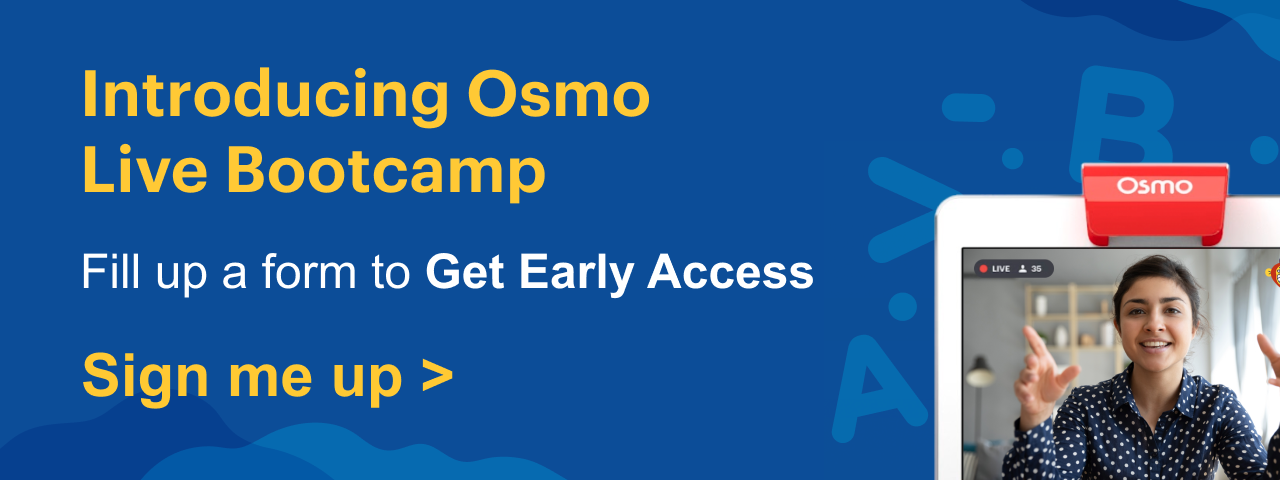Learning numbers is essential to help kids learn math. By the time they reach kindergarten, children should be able to recognize, read and write numbers. So, start by introducing kids to the number 1 with number 1 worksheets. These free printable number 1 tracing, adding, and subtracting worksheets will help kids learn how to trace and write the number clearly.

Number 1 worksheets are a great tool to help children learn to recognize and write number one. Additionally, these numbers worksheets introduces children to basic math concepts too like addition and subtraction.

## Free Printable Number 1 Worksheets

Here are a few worksheets for kids to learn numbers in a different way.

### Number 1 Tracing Worksheets for Toddlers

Help your toddler learn to recognize, trace and color the number 1 using these free number 1 tracing worksheets. These worksheets for kids help them learn to write number 1 in numerical form and in words. Learning to write number 1 in numerical or in words help them develop their writing skills.

### Number 1 Pre K Worksheets

Kids in pre K can recognize a few numbers and count too. Put your preschoolers to the test and ask them to find, circle and color all the number 1s in this worksheet. Check out number 1 worksheet for kids in preschool to find and color the number for gaining mathematical knowledge.

Also, refer to the Kindergarten Number Line.

### Kindergarten Number 1 Addition Worksheets

Kids in kindergarten are more confident with numbers and can count too. They are also taught basic math concepts like addition and subtraction. Help your child learn to add and subtract the number 1 using this worksheet.

### Kindergarten Number 1 Subtraction Worksheets

Help your kindergarten children practice subtraction of numbers with one digit using the worksheet given below. Kids can easily subtract the given numbers with one digit, for example, 15 – 1 = 14. Similarly, they can practice subtraction for the equations given below. This is the best way to introduce number 1 to kids for effective learning experience.

Also check out the 1 times table for kids.

## Benefits of Using Worksheets To Teach Number 1 for Kids

While kids learn numbers easily, it is important to help them retain the information in their memory. Most kids tend to forget what they’ve learnt as quickly as they move on to the next topic. Making children solve number worksheets has a lot of benefits.

Here are some advantages of making children work on number 1 worksheets:

1. Better memory retention: Solving number worksheets is a great way to reinforce number lessons for kids. They learn to write as they trace and color the numbers and words. This helps them retain the knowledge in their memory for a long time.
2. Fine motor skills: Tracing and coloring on these worksheets also builds the child’s fine motor skills. These activities have been proven to build strength in the muscles of a child’s arms. It also improves their hand-eye coordination skills.
3. Basic math skills: Along with learning how to write numbers, children learn other basic math skills when they solve these worksheets. These worksheets help children learn how to count and add and subtract the number 1.

For more worksheets, math games for kids, and other learning activities, check out Osmo’s kids learning section.

## Frequently Asked Questions on Number 1 Worksheets

### What are the different types of fun number 1 worksheets?

The different types of fun number 1 worksheets are number 1 worksheets for kindergarten, preschool, and pre-K, addition number 1 worksheets, subtraction number 1 worksheets, multiplication number 1 worksheets, and division number 1 worksheets.

### Why should kids be given number 1 worksheets?

Kids must be introduced to the number 1 worksheets because these worksheets will help them to understand the basic concepts of Numbers and their properties in a fun yet simple way. They also improve their decision-making, problem-solving, and overall cognitive skills.

 Kids Learning Related Links Christmas Word That Starts With B Egg Drop Experiment Words That Start With Pre Easy Math Riddles For Kids Baby Letter Matching Game First Birthday Gift Ideas Interactive Math Games For Grade 1 Best Gifts For 2 Year Olds